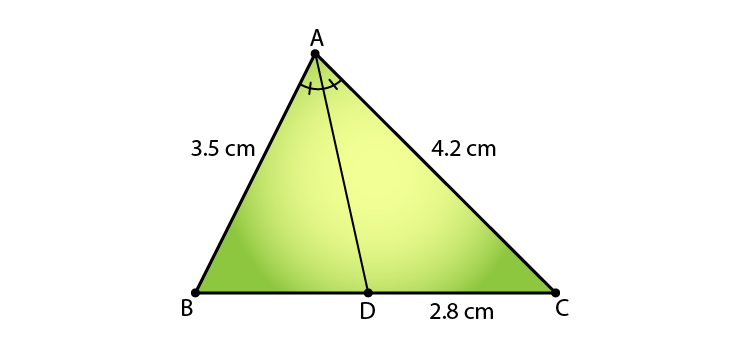Guru

# In a ∆ABC, AD is the bisector of ∠A, meeting side BC at D.If AB = 3.5 cm, AC = 4.2 cm and DC = 2.8 cm, find BD

• 0

Question taken from RD sharma
Class 10th
Chapter no. 4
Chapter name:- Triangles
Exercise :- 4.3
This is very basic and important questions.

In this question we have a ∆ABC, AD is the bisector of ∠A, meeting side BC at D.

Also sides measurements are given AB = 3.5 cm, AC = 4.2 cm and DC = 2.8 cm,

Now have to find BD

Understanding and learning CBSE maths
RD sharma, DHANPAT RAI publication

Share

1. Given: Δ ABC and AD bisects ∠A, meeting side BC at D. And AB = 3.5 cm, AC = 4.2 cm, and DC = 2.8 cm.

Required to find: BDSince, AD is the bisector of ∠ A meeting side BC at D in Δ ABC

⇒ AB/ AC = BD/ DC

3.5/ 4.2 = BD/ 2.8

4.2 x BD = 3.5 x 2.8

BD = 7/3

∴ BD = 2.3 cm

• 0